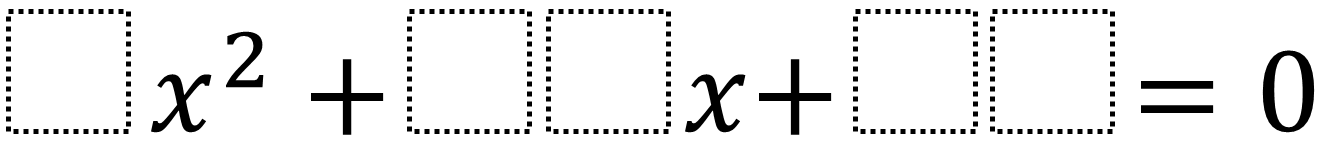# Factoring Quadratics 2 – Fraction Solution

Directions: Using the digits 0 to 9 at most one time each, fill in the boxes to so that at least one of the solutions is a fraction.### Hint

How could you find the equation if you already knew the solutions?

There are many answers including 9x^2 + 28x + 3 which has solutions of -1/9 and -3.

Source: Daniel Luevanos

## Difference of Squares and Sum of Cubes

Directions: Using the digits 0 to 9 at most one time each, place a digit …

### One comment

1.Hi there. Maybe rational number is a better word that a fraction. I was expecting my students to find numbers so that at least one solution was rational but some students created a quadratic so that, for example, (7+ sqrt3)/4 was one solution. Technically it is a fraction but not what I was expecting.